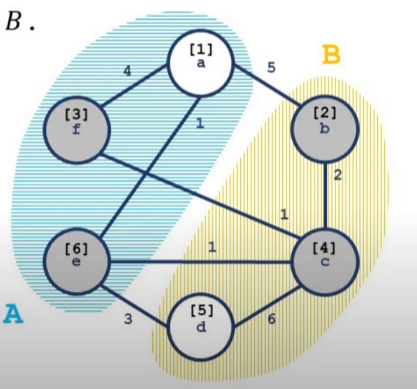# Stoer–Wagner 算法

## 定义

（其实是网络流部分有关割的定义与维基百科不符，只是由于一般接触到的割都是「有源汇的最小割问题」，因此这个概念也就约定俗成了。）

## Stoer–Wagner 算法

### 引入

Stoer–Wagner 算法在 1995 年由Mechthild StoerFrank Wagner提出，是一种通过 递归 的方式来解决 无向正权图 上的全局最小割问题的算法。

### 过程

1. 在图中任意指定两点，并且以这两点作为源汇点求出图最小割（定义为cut of phase），更新当前答案。
2. 「合并」点，如果图大于，则回到第一步。
3. 输出所有cut of phase的最小值。

### S-T 最小割的求法

（显然不是网络流。）（若，则）。

### 证明Lemma 1并且联立可得：P5632【模板】Stoer–Wagner 算法
  1 2 3 4 5 6 7 8 9 10 11 12 13 14 15 16 17 18 19 20 21 22 23 24 25 26 27 28 29 30 31 32 33 34 35 36 37 38 39 40 41 42 43 44 45 46 47 48 49 50 51 52 53 54 55 56 57 58 59 60 61 62 63 64 65 66 67 68 69 70 71 72 73 #include using namespace std; const int N = 601; int fa[N], siz[N], edge[N][N]; int find(int x) { return fa[x] == x ? x : fa[x] = find(fa[x]); } int dist[N], vis[N], bin[N]; int n, m; int contract(int &s, int &t) { // Find s,t memset(dist, 0, sizeof(dist)); memset(vis, false, sizeof(vis)); int i, j, k, mincut, maxc; for (i = 1; i <= n; i++) { k = -1; maxc = -1; for (j = 1; j <= n; j++) if (!bin[j] && !vis[j] && dist[j] > maxc) { k = j; maxc = dist[j]; } if (k == -1) return mincut; s = t; t = k; mincut = maxc; vis[k] = true; for (j = 1; j <= n; j++) if (!bin[j] && !vis[j]) dist[j] += edge[k][j]; } return mincut; } const int inf = 0x3f3f3f3f; int Stoer_Wagner() { int mincut, i, j, s, t, ans; for (mincut = inf, i = 1; i < n; i++) { ans = contract(s, t); bin[t] = true; if (mincut > ans) mincut = ans; if (mincut == 0) return 0; for (j = 1; j <= n; j++) if (!bin[j]) edge[s][j] = (edge[j][s] += edge[j][t]); } return mincut; } int main() { ios::sync_with_stdio(0), cin.tie(0); cin >> n >> m; if (m < n - 1) { cout << 0; return 0; } for (int i = 1; i <= n; ++i) fa[i] = i, siz[i] = 1; for (int i = 1, u, v, w; i <= m; ++i) { cin >> u >> v >> w; int fu = find(u), fv = find(v); if (fu != fv) { if (siz[fu] > siz[fv]) swap(fu, fv); fa[fu] = fv, siz[fv] += siz[fu]; } edge[u][v] += w, edge[v][u] += w; } int fr = find(1); if (siz[fr] != n) { cout << 0; return 0; } cout << Stoer_Wagner(); return 0; } 

### 复杂度分析与优化

contract操作的复杂度为（实际测试中开 O2 还要卡评测波动才能过。）